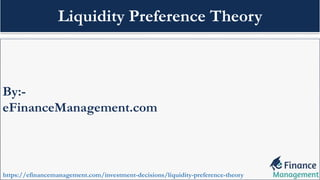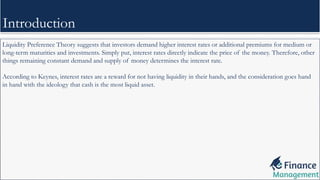Diese Präsentation wurde erfolgreich gemeldet.

# Liquidity Preference Theory

Anzeige
Anzeige
Anzeige
Anzeige
Anzeige
Anzeige
Anzeige
Anzeige
Anzeige
Anzeige
Anzeige×

1 von 9 Anzeige

# Liquidity Preference Theory

Liquidity Preference Theory suggests that investors demand higher interest rates or additional premiums for medium or long-term maturities and investments. Simply put, interest rates directly indicate the price of the money.
https://efinancemanagement.com/investment-decisions/liquidity-preference-theory

Liquidity Preference Theory suggests that investors demand higher interest rates or additional premiums for medium or long-term maturities and investments. Simply put, interest rates directly indicate the price of the money.
https://efinancemanagement.com/investment-decisions/liquidity-preference-theory

Anzeige
Anzeige

### Liquidity Preference Theory

1. 1. By:- eFinanceManagement.com https://efinancemanagement.com/investment-decisions/liquidity-preference-theory Liquidity Preference Theory
2. 2. 1. Introduction 2. Demand for Money 3. Total Demand 4. Supply for Money 5. Determination of Interest Rate 6. Limitations 7. Reference Content
3. 3. Liquidity Preference Theory suggests that investors demand higher interest rates or additional premiums for medium or long-term maturities and investments. Simply put, interest rates directly indicate the price of the money. Therefore, other things remaining constant demand and supply of money determines the interest rate. According to Keynes, interest rates are a reward for not having liquidity in their hands, and the consideration goes hand in hand with the ideology that cash is the most liquid asset. Introduction
4. 4. There are 3 main reasons (motives) for demand for money. These motives are as follows: 1. Transaction Motives: In order to satisfy all daily needs, the individual requires money with a transaction motive. Demand through transaction motive is inelastic to interest rates and elastic to income level. Expression of transaction motive is: T = f (Y) 2. Precautionary Motives: This is the second motive. Because the future is uncertain and there could be sudden natural or man-made disasters, Individuals demand money with a precautionary motive for protection against uncertain future. Expression of precautionary motive is: P = f (Y) 3. Speculative Motive: Individuals demand money by taking into account fluctuations in interest rates or bond prices. If interest rates are lower or bond prices are lower, there is a high demand for money with speculative motives and vice versa. Expression of speculative motive is: S = f (R) Demand for Money
5. 5. The aggregate demand for money or the function of liquidity preference would be the sum of all three motives transaction, precautionary and speculative. TD = T + P + S TD = f (Y) + f (Y) = f (R) Total Demand = f (Y, R) Total Demand
6. 6. According to John Maynard Keynes, the supply of money is largely fixed and determined by the country’s central bank. Hence, according to the theory of liquidity preference, the supply is perfectly inelastic and graphically represents a straight vertical line. Supply for Money
7. 7. The interest rate is determined at the point where the demand for money is equal to the supply of money. The TD curve is downward sloping from left to right. This is due to the opposite link between the demand for money and the interest rate. Whereas the Supply (SP) curve is perfectly inelastic and represents a vertical straight line. Determination of Interest Rate
8. 8. The limitations of Liquidity Preference Theory are as follows: • It assumes that the employment rate is constant. • It assumes a certain level of income. • It completely ignores the scenario of receiving interest benefits for some funds and receiving liquidity benefits for remaining funds. • Ignores that different interest rates exist in different markets at the same time. • The savings done by individuals is not considered Limitations
9. 9. Reference To know more about it, click on the link given below: https://efinancemanagement.com/investment-decisions/liquidity-preference-theory